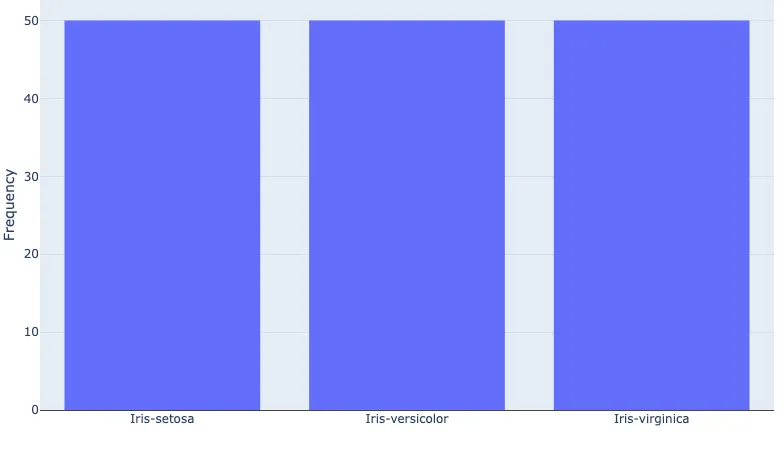## Data Analysis with Rust Notebooks

A practical book on Data Analysis with Rust Notebooks that teaches you the concepts and how they’re implemented in practice.

Get the book## Preamble

``````:dep darn = {version = "0.3.4"}
:dep ndarray = {version = "0.13.1"}
:dep ndarray-csv = {version = "0.4.1"}
:dep ureq = {version = "0.11.4"}
:dep itertools = {version = "0.9.0"}
:dep plotly = {version = "0.4.0"}
extern crate csv;
extern crate itertools;

use std::io::prelude::*;
use std::fs::*;
use ndarray::prelude::*;
use std::str::FromStr;
use itertools::Itertools;
use plotly::{Plot, Bar, Layout};
use plotly::common::{Mode, Title};
use plotly::layout::{Axis};
use std::collections::HashMap;
``````

## Introduction

In this section, we're going to take a look at some approaches to determining the unique elements in a column of data and their frequency. This is a common task which we may often apply to categorical data, and it can easily give us a good idea of the balance of categorical values across our dataset.

If you're familiar with Python and NumPy, you may have encountered the `numpy.unique()`function that returns a list of sorted unique elements of an array. In more recent versions of NumPy, the `numpy.unique()` function also takes a parameter named `return_counts`, which when set to `True` will also return the number of times each unique element appears in the array.

Let's see how we can do the same for our `ndarray::Array2`.

We will continue using the Iris Flower dataset, so we need to load it into our raw string array first.

``````let file_name = "Iris.csv";

let res = ureq::get("https://datacrayon.com/datasets/Iris.csv").call().into_string()?;

let mut file = File::create(file_name)?;
file.write_all(res.as_bytes());
remove_file(file_name)?;

let data: Array2<String>= rdr.deserialize_array2_dynamic().unwrap();
let mut headers : Vec<String> = Vec::new();

};
``````

### Moving Data to Typed Arrays

We need to convert from String to the desired type, and move our data over to the typed arrays.

``````let mut features: Array2::<f32> =  Array2::<f32>::zeros((data.shape(),0));

for &f in [1, 2, 3, 4].iter() {
features = ndarray::stack![Axis(1), features,
data.column(f as usize)
.mapv(|elem| f32::from_str(&elem).unwrap())
.insert_axis(Axis(1))];
};

let labels: Array1::<String> = data.column(5).to_owned();
``````

We will only be using our species labels, stored in `labels`, throughout the rest of this section.

## Unique Elements

We can get the unique elements in our array using the `unique()` function provided by the `itertools` crate.

Return an iterator adaptor that filters out elements that have already been produced once during the iteration. Duplicates are detected using hash and equality.

We can then iterate through this and output the elements to the output cell.

``````for i in labels.iter().unique() {
println!("{}",i);
};
``````

We can also use the `.format()` function on `.unique()` to print out our unique elements.

``````labels.iter().unique().format("\n")
``````
```Iris-setosa
Iris-versicolor
Iris-virginica
```
```"Iris-setosa"
"Iris-versicolor"
"Iris-virginica"```

However, we may want to store these in a vector to use later too. Let's store them in `unique_elements`.

``````let unique_elements = labels.iter().cloned().unique().collect_vec();

unique_elements
``````
`["Iris-setosa", "Iris-versicolor", "Iris-virginica"]`

## Count of Unique Elements

In this case it's easy to see we have three unique elements, but if the list is too long or we wanted to store the value for later use, we can use the `len()` function on our vector containing the unique elements.

``````unique_elements.len()
``````
`3`

We could also get the count from our iterator.

``````labels.iter().unique().count()
``````
`3`

## Frequency of Unique Elements

To find the frequency of a specific string we can use `filter()` and then `count()`.

``````labels.iter().filter(|&elem| *elem == "Iris-virginica").count()
``````
`50`

We may also want to find the frequency of every unique element and store it in a vector. We'll call this `unique_frequency`.

``````let mut unique_frequency = Vec::<usize>::new();
``````

We can populate this by iterating through the unique elements and use the strings to find the frequency using the `filter()` approach above.

``````for unique_elem in unique_elements.iter() {
unique_frequency.push(labels.iter().filter(|&elem| elem == unique_elem).count());
};

unique_frequency
``````
`[50, 50, 50]`

## Visualise the Frequency of Unique Elements

It's useful to visualise the frequency of elements in our array, especially if we're interested in looking at the balance in our dataset. We can do this with a `Bar` plot using `Plotly`.

``````let layout = Layout::new()
.yaxis(Axis::new().title(Title::new("Frequency")));

let freq_bars = Bar::new(unique_elements, unique_frequency);

let mut plot = Plot::new();

plot.set_layout(layout);

darn::show_plot(plot)
``````

## Unique Elements and their Frequency with Hashmaps

We can do something similar with a `HashMap`. First we'll define a variable of type `HashMap<String, i32>`, where the `String` part will be the unique element, and the `i32` will be the frequency.

``````let mut value_counts : HashMap<String, i32> = HashMap::new();
``````

We can then populate this by iterating through our original `labels` array.

``````for item in labels.iter() {
*value_counts.entry(String::from(item)).or_insert(0) += 1;
};
``````

Printing out the results, we can see that `value_counts` now contains all the information that we're after.

``````println!("{:#?}", value_counts);
``````
```{
"Iris-virginica": 50,
"Iris-setosa": 50,
"Iris-versicolor": 50,
}
```

We can also get these values directly by key.

``````value_counts.get("Iris-versicolor").unwrap()
``````
`50`

However, you will need to map this `HashMap` to separate vectors if you want to use it for plotting with Plotly.

## Conclusion

In this section, we've demonstrated a few approaches to identifying the unique elements in an array, counting the number of unique elements, and the frequency of these unique elements. We also visualised the frequency of our unique elements which is useful during exploratory data analysis.

## Data Analysis with Rust Notebooks

A practical book on Data Analysis with Rust Notebooks that teaches you the concepts and how they’re implemented in practice.

Get the book

## ISBN

978-1-915907-10-3

## Cite

Rostami, S. (2020). Data Analysis with Rust Notebooks. Polyra Publishing.